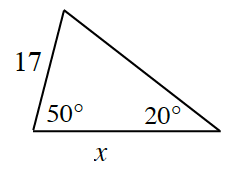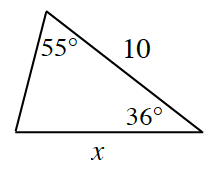### Home > INT3 > Chapter 10 > Lesson 10.1.1 > Problem10-12

10-12.

What is the value of $x$ in each diagram below?

1.Recall the Triangle Angle Sum Theorem and solve for the missing angle measurement.

Use the Law of Sines to solve for $x$.

$x ≈ 46.71$

1.See hints from part (a).